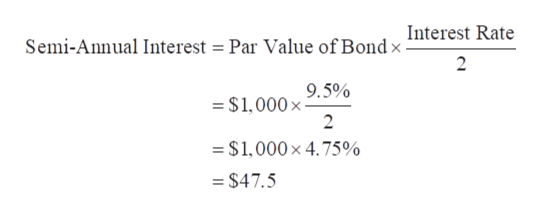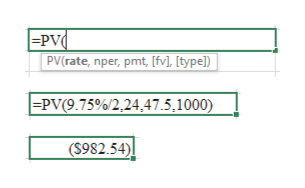What is the price of a 12 year bond paying semiannual interest at a rate of 9.5% with a yield to maturity of 9.75%?  Assume par is \$1,000.

Question

What is the price of a 12 year bond paying semiannual interest at a rate of 9.5% with a yield to maturity of 9.75%?  Assume par is \$1,000.

Step 1

Par Value of Bond = \$1,000

Interest Rate = 9.5%

Type of Interest = Semi-annual

Calculation of semi-annual interest payment:help_outlineImage TranscriptioncloseSemi-Annual Interest = Par Value of Bond x Interest Rate 2 - \$1,0009.5% 2 =\$1,000 x 4.75% = \$47.5 fullscreen
Step 2

Par Value of Bond (fv) = \$1,000

Semi-annual Interest (pmt) = \$47.5

Time Period = 12 years

Number of Payments per year = 2

Time to Maturity (nper) = 24

Yield to Maturity (rate) = 9.75%

&nbs...help_outlineImage Transcriptionclose|=PV¢ PV(rate, nper, pmt, [fv], [type]) |=PV(9.75%/2,24,47.5,1000) (S982.54) fullscreen

Want to see the full answer?

See Solution

Want to see this answer and more?

Our solutions are written by experts, many with advanced degrees, and available 24/7

See Solution
Tagged in